## Class 11 Important Questions for Chemistry – States of Matter

NCERT Exemplar Class 11 Chemistry is very important resource for students preparing for XI Board Examination. Here we have provided NCERT Exemplar Problems Solutions along with NCERT Exemplar Problems Class 11.

Question from very important topics are covered by NCERT Exemplar Class 11. You also get idea about the type of questions and method to answer in your Class 11th examination.

for Class 11 Chemistry Notes

Here you can get Class 11 Important Questions Chemistry based on NCERT Text book for Class XI. Chemistry Class 11 Important Questions are very helpful to score high marks in board exams. Here we have covered Important Questions on States of Matter for Class 11 Chemistry subject.

Chemistry Important Questions Class 11 are given below.

### Multiple Choice Questions (Type-I)

1. A person living in Shimla observed that cooking food without using pressure cooker takes more time. The reason for this observation is that at high altitude:
• (i) pressure increases
• (ii) temperature decreases
• (iii) pressure decreases
• (iv) temperature increases
2. Which of the following property of water can be used to explain the spherical shape of rain droplets?
• (i) viscosity
• (ii) surface tension
• (iii) critical phenomena
• (iv) pressure
3. A plot of volume (V ) versus temperature (T ) for a gas at constant pressure is a straight line passing through the origin. The plots at different values of pressure are shown in Fig. 5.1. Which of the following order of pressure is correct for this gas?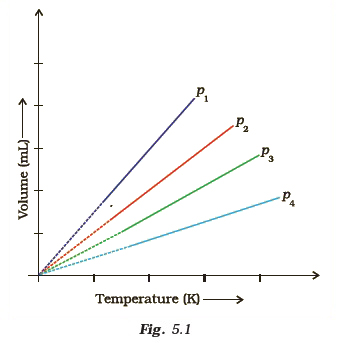• (i) p1 > p2 > p3 > p4
• (ii) p1 = p2 = p3 = p4
• (iii) p1 < p2 < p3 < p4
• (iv) p1 < p2 = p3 < p4
4. The interaction energy of London force is inversely proportional to sixth power of the distance between two interacting particles but their magnitude depends upon
• (i) charge of interacting particles
• (ii) mass of interacting particles
• (iii) polarisability of interacting particles
• (iv) strength of permanent dipoles in the particles.
5. Dipole-dipole forces act between the molecules possessing permanent dipole. Ends of dipoles possess ‘partial charges’. The partial charge is
• (i) more than unit electronic charge
• (ii) equal to unit electronic charge
• (iii) less than unit electronic charge
• (iv) double the unit electronic charge
6. The pressure of a 1:4 mixture of dihydrogen and dioxygen enclosed in a vessel is one atmosphere. What would be the partial pressure of dioxygen?
• (i) 0.8 × 105 atm
• (ii) 0.008 Nm–2
• (iii) 8 × 104 Nm–2
• (iv) 0.25 atm
7. As the temperature increases, average kinetic energy of molecules increases. What would be the effect of increase of temperature on pressure provided the volume is constant?
• (i) increases
• (ii) decreases
• (iii) remains same
• (iv) becomes half
8. Gases possess characteristic critical temperature which depends upon the magnitude of intermolecular forces between the particles. Following are the critical temperatures of some gases.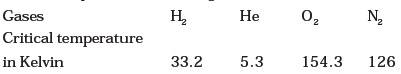From the above data what would be the order of liquefaction of these gases? Start writing the order from the gas liquefying first
• (i) H2, He, O2, N2
• (ii) He, O2, H2, N2
• (iii) N2, O2, He, H2
• (iv) O2, N2, H2, He
9. What is SI unit of viscosity coefficient (η)?
• (i) Pascal
• (ii) Nsm–2
• (iii) km–2 s
• (iv) N m–2
10. Atmospheric pressures recorded in different cities are as follows:Consider the above data and mark the place at which liquid will boil first.
• (i) Shimla
• (ii) Bangalore
• (iii) Delhi
• (iv) Mumbai
11. Which curve in Fig. 5.2 represents the curve of ideal gas?• (i) B only
• (ii) C and D only
• (iii) E and F only
• (iv) A and B only
12. Increase in kinetic energy can overcome intermolecular forces of attraction. How will the viscosity of liquid be affected by the increase intemperature?
• (i) Increase
• (ii) No effect
• (iii) Decrease
• (iv) No regular pattern will be followed
13. How does the surface tension of a liquid vary with increase in temperature?
• (i) Remains same
• (ii) Decreases
• (iii) Increases
• (iv) No regular pattern is followed

### Multiple Choice Questions (Type-II)

In the following questions two or more options may be correct.

1. With regard to the gaseous state of matter which of the following statements are correct?
• (i) Complete order of molecules
• (ii) Complete disorder of molecules
• (iii) Random motion of molecules
• (iv) Fixed position of molecules
2. Which of the following figures does not represent 1 mole of dioxygen gas at STP?
• (i) 16 grams of gas
• (ii) 22.7 litres of gas
• (iii) 6.022 × 1023 dioxygen molecules
• (iv) 11.2 litres of gas
3. Under which of the following two conditions applied together, a gas deviates most from the ideal behaviour?
• (i) Low pressure
• (ii) High pressure
• (iii) Low temperature
• (iv) High temperature
4. Which of the following changes decrease the vapour pressure of water kept in a sealed vessel?
• (i) Decreasing the quantity of water
• (ii) Adding salt to water
• (iii) Decreasing the volume of the vessel to one-half
• (iv) Decreasing the temperature of water

### Short Answer Type Questions

1. If 1 gram of each of the following gases are taken at STP, which of the gases will occupy (a) greatest volume and (b) smallest volume? CO, H2O, CH4, NO
2. Physical properties of ice, water and steam are very different. What is the chemical composition of water in all the three states.
3. The behaviour of matter in different states is governed by various physical laws. According to you what are the factors that determine the state of matter?
4. Use the information and data given below to answer the questions (a) to (c):
• Stronger intermolecular forces result in higher boiling point.
• Strength of London forces increases with the number of electrons in the molecule.
• Boiling point of HF, HCl, HBr and HI are 293 K, 189 K, 206 K and 238 K respectively.
• (a) Which type of intermolecular forces are present in the molecules HF, HCl, HBr and HI?
• (b) Looking at the trend of boiling points of HCl, HBr and HI, explain out of dipole-dipole interaction and London interaction, which one is predominant here.
• (c) Why is boiling point of hydrogen fluoride highest while that of hydrogen chloride lowest?
5. What will be the molar volume of nitrogen and argon at 273.15K and 1 atm?
6. A gas that follows Boyle’s law, Charle’s law and Avogadro’s law is called an ideal gas. Under what conditions a real gas would behave ideally?
7. Two different gases ‘A’ and ‘B’ are filled in separate containers of equal capacity under the same conditions of temperature and pressure. On increasing the pressure slightly the gas ‘A’ liquefies but gas B does not liquify even on applying high pressure until it is cooled. Explain this phenomenon.
8. Value of universal gas constant (R) is same for all gases. What is its physical significance?
9. One of the assumptions of kinetic theory of gases states that “there is no force of attraction between the molecules of a gas.” How far is this statement correct?
Is it possible to liquefy an ideal gas? Explain.
10. The magnitude of surface tension of liquid depends on the attractive forces between the molecules. Arrange the following in increasing order of surface tension :
water, alcohol (C2H5OH) and hexane [CH3(CH2)4CH3)].
11. Pressure exerted by saturated water vapour is called aqueous tension. What correction term will you apply to the total pressure to obtain pressure of dry gas?
12. Name the energy which arises due to motion of atoms or molecules in a body. How is this energy affected when the temperature is increased?
13. Name two intermolecular forces that exist between HF molecules in liquid state.
14. One of the assumptions of kinetic theory of gases is that there is no force of attraction between the molecules of a gas.
State and explain the evidence that shows that the assumption is not applicable for real gases.
15. Compressibility factor, Z, of a gas is given as
Z = (pV/nRT)
(i) What is the value of Z for an ideal gas?
(ii) For real gas what will be the effect on value of Z above Boyle’s temperature?
16. The critical temperature (Tc) and critical pressure ( pc) of CO2 are 30.98°C and 73 atm respectively. Can CO2 (g) be liquefied at 32°C and 80 atm pressure?
17. For real gases the relation between p, V and T is given by van der Waals equation: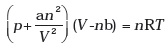where ‘a’ and ‘b’ are van der Waals constants, ‘nb’ is approximately equal to the total volume of the molecules of a gas.
‘a’ is the measure of magnitude of intermolecular attraction.
(i) Arrange the following gases in the increasing order of ‘b’. Give reason.
O2, CO2, H2, He
(ii) Arrange the following gases in the decreasing order of magnitude of ‘a’.
Give reason.
CH4, O2, H2
18. The relation between pressure exerted by an ideal gas (pideal) and observed pressure (preal) is given by the equation
Pideal = Preal + (an2/V2)
If pressure is taken in Nm–2, number of moles in mol and volume in m3, Calculate the unit of ‘a’.
What will be the unit of ‘a’ when pressure is in atmosphere and volume in dm3?
19. Name two phenomena that can be explained on the basis of surface tension.
20. Viscosity of a liquid arises due to strong intermolecular forces existing between the molecules. Stronger the intermolecular forces, greater is the viscosity. Name the intermolecular forces existing in the following liquids and arrange them in the increasing order of their viscosities. Also give reason for the assigned order in one line.
Water, hexane (CH3CH2CH2CH2CH2CH3), glycerine (CH2OH CH(OH) CH2OH)
21. Explain the effect of increasing the temperature of a liquid, on intermolecular forces operating between its particles, what will happen to the viscosity of a liquid if its temperature is increased?
22. The variation of pressure with volume of the gas at different temperatures can be graphically represented as shown in Fig. 5.3.
On the basis of this graph answer the following questions.• (i) How will the volume of a gas change if its pressure is increased at constant temperature?
• (ii) At a constant pressure, how will the volume of a gas change if the temperature is increased from 200K to 400K?
23. Pressure versus volume graph for a real gas and an ideal gas are shown in Fig. 5.4. Answer the following questions on the basis of this graph.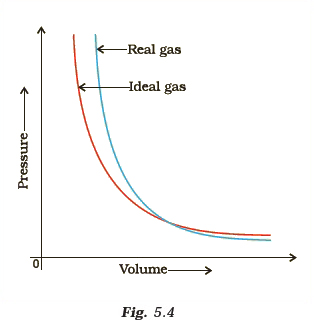• (i) Interpret the behaviour of real gas with respect to ideal gas at low pressure.
• (ii) Interpret the behaviour of real gas with respect to ideal gas at high pressure.
• (iii) Mark the pressure and volume by drawing a line at the point where real gas behaves as an ideal gas.

### Matching Type Questions

1. Match the graphs between the following variables with their names :2. Match the following gas laws with the equation representing them.3. Match the following graphs of ideal gas with their co-ordinates :### Assertion and Reason Type Questions

In the following questions a statement of Assertion (A) followed by a statement of Reason (R) is given. Choose the correct option out of the choices given below each question.

1. Assertion (A): Three states of matter are the result of balance between intermolecular forces and thermal energy of the molecules.
Reason (R): Intermolecular forces tend to keep the molecules together but thermal energy of molecules tends to keep them apart.
• (i) Both A and R are true and R is the correct explanation of A.
• (ii) Both A and R are true but R is not the correct explanation of A.
• (iii) A is true but R is false.
• (iv) A is false but R is true.
2. Assertion (A): At constant temperature, pV vs V plot for real gases is not a straight line.
Reason (R) : At high pressure all gases have Z > 1 but at intermediate pressure most gases have Z < 1.
• (i) Both A and R are true and R is the correct explanation of A.
• (ii) Both A and R are true but R is not the correct explanation of A.
• (iii) A is true but R is false.
• (iv) A is false but R is true.
3. Assertion (A): The temperature at which vapour pressure of a liquid is equal to the external pressure is called boiling temperature.
Reason (R) : At high altitude atmospheric pressure is high.
• (i) Both A and R are true and R is the correct explanation of A.
• (ii) Both A and R are true but R is not the correct explanation of A.
• (iii) A is true but R is false.
• (iv) A is false but R is true.
4. Assertion (A): Gases do not liquefy above their critical temperature, even on applying high pressure.
Reason (R) : Above critical temperature, the molecular speed is high and intermolecular attractions cannot hold the molecules together because they escape because of high speed.
• (i) Both A and R are true and R is the correct explanation of A.
• (ii) Both A and R are true but R is not the correct explanation of A.
• (iii) A is true but R is false.
• (iv) A is false but R is true.
5. Assertion (A): At critical temperature liquid passes into gaseous state imperceptibly and continuously.
Reason (R) : The density of liquid and gaseous phase is equal to critical temperature.
• (i) Both A and R are true and R is the correct explanation of A.
• (ii) Both A and R are true but R is not the correct explanation of A.
• (iii) A is true but R is false.
• (iv) A is false but R is true.
6. Assertion (A): Liquids tend to have maximum number of molecules at their surface.
Reason (R) : Small liquid drops have spherical shape.
• (i) Both A and R are true and R is the correct explanation of A.
• (ii) Both A and R are true but R is not the correct explanation of A.
• (iii) A is true but R is false.
• (iv) A is false but R is true.

### Long Answer Type Questions

1. Isotherms of carbon dioxide at various temperatures are represented in Fig. 5.5. Answer the following questions based on this figure.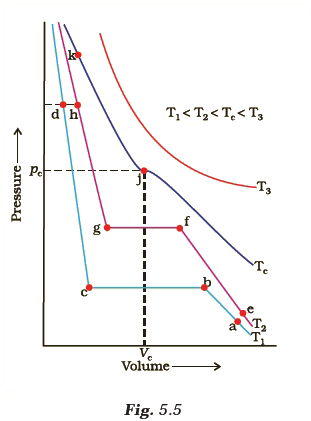• (i) In which state will CO2 exist between the points a and b at temperature T1?
• (ii) At what point will CO2 start liquefying when temperature is T1?
• (iii) At what point will CO2 be completely liquefied when temperature is T2.
• (iv) Will condensation take place when the temperature is T3.
• (v) What portion of the isotherm at T1 represent liquid and gaseous CO2 at equilibrium?
2. The variation of vapour pressure of different liquids with temperature is shown in Fig. 5.6.• (i) Calculate graphically boiling points of liquids A and B.
• (ii) If we take liquid C in a closed vessel and heat it continuously. At what temperature will it boil?
• (iii) At high altitude, atmospheric pressure is low (say 60 mm Hg). At what temperature liquid D boils?
• (iv) Pressure cooker is used for cooking food at hill station. Explain in terms of vapour pressure why is it so?
3. Why does the boundary between liquid phase and gaseous phase disappear on heating a liquid upto critical temperature in a closed vessel? In this situation what will be the state of the substance?
4. Why does sharp glass edge become smooth on heating it upto its melting point in a flame? Explain which property of liquids is responsible for this phenomenon.
5. Explain the term ‘laminar flow’. Is the velocity of molecules same in all the layers in laminar flow? Explain your answer.
6. Isotherms of carbon dioxide gas are shown in Fig. 5.7. Mark a path for changing gas into liquid such that only one phase (i.e., either a gas or a liquid) exists at any time during the change. Explain how the temperature, volume and pressure should be changed to carry out the change.« Previous Next »

## Answers to Multiple Choice Questions

 MCQ (Type-I) Q.No. Answer Q.No. Answer Q.No. Answer Q.No. Answer Q.No. Answer Q.No. Answer 1 (iii) 2 (ii) 3 (iii) 4 (iii) 5 (iii) 6 (iii) 7 (i) 8 (iv) 9 (ii) 10 (i) 11 (i) 12 (iii) 13 (ii)
 MCQ (Type-II) Q.No. Answer Q.No. Answer Q.No. Answer 1 (ii), (iii) 2 (i), (iv) 3 (ii), (iii) 4 (ii), (iv)